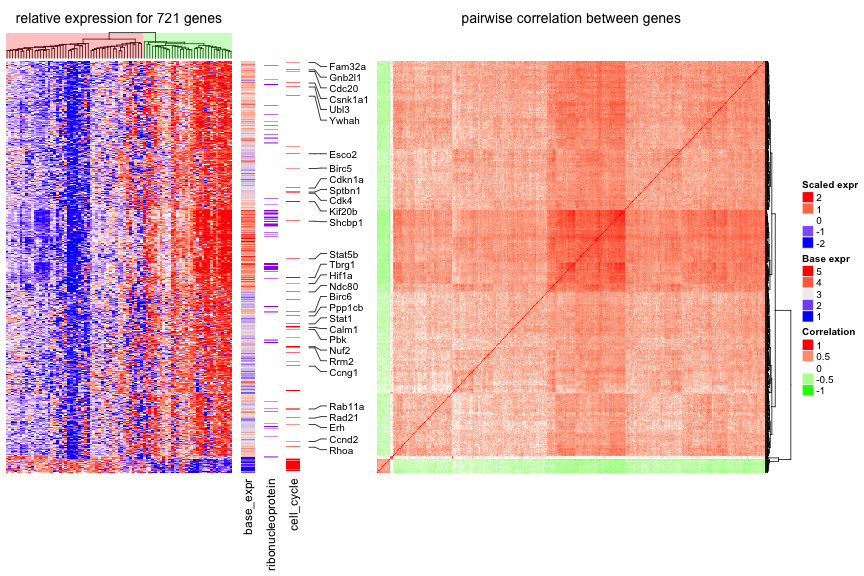Supplementary S2. Visualize Cell Heterogeneity from Single Cell RNASeq

Author: Zuguang Gu ( z.gu@dkfz.de )

Date: 2016-05-13

To successfully run this example, ComplexHeatmap >= 1.10.1. is required. The newest version can be obtained by:

library(devtools)
install_github("jokergoo/Complexheatmap")

library(circlize)
library(ComplexHeatmap)
library(GetoptLong)

In this supplementary, single cell RNA-Seq data for mouse T-cells is visualized to show the heterogeneity of cells. The data (mouse_scRNAseq_corrected.txt) is from Buettner et al., 2015, supplementary data 1, sheet “Cell-cycle corrected gene expr”.

In following code, duplicated genes are removed.

expr = expr[!duplicated(expr[]), ]
rownames(expr) = expr[]
expr = expr[-1]
expr = as.matrix(expr)

Genes that are not expressed in more than half of the cells are filtered out.

expr = expr[apply(expr, 1, function(x) sum(x > 0)/length(x) > 0.5), , drop = FALSE]

The get_correlated_variable_rows() function is defined here. It extracts signature genes that are variably expressed between cells and correlate to other genes.

get_correlated_variable_genes = function(mat, n = nrow(mat), cor_cutoff = 0, n_cutoff = 0) {
ind = order(apply(mat, 1, function(x) {
q = quantile(x, c(0.1, 0.9))
x = x[x < q & x > q]
var(x)/mean(x)
}), decreasing = TRUE)[1:n]
mat2 = mat[ind, , drop = FALSE]
dt = cor(t(mat2), method = "spearman")
diag(dt) = 0
dt[abs(dt) < cor_cutoff] = 0
dt[dt < 0] = -1
dt[dt > 0] = 1

i = colSums(abs(dt)) > n_cutoff

mat3 = mat2[i, ,drop = FALSE]
return(mat3)
}

Signature genes are defined as a list of genes where each gene correlates to more than 20 genes with an absolute correlation larger than 0.5.

mat2 contains expression values scaled per gene, which means it contains relative expression across cells for every gene. Since single cell RNASeq data is highly variable and outliers are frequent, gene expression is only scaled within the 10th and 90th quantiles.

mat = get_correlated_variable_genes(expr, cor_cutoff = 0.5, n_cutoff = 20)
mat2 = t(apply(mat, 1, function(x) {
q10 = quantile(x, 0.1)
q90 = quantile(x, 0.9)
x[x < q10] = q10
x[x > q90] = q90
scale(x)
}))
colnames(mat2) = colnames(mat)

Load cell cycle genes and ribonucleoprotein genes. The cell cycle gene list is from Buettner et al., 2015, supplementary table 1, sheet “Union of Cyclebase and GO genes”. Ribonucleoprotein genes are from GO:0030529. Gene list are stored in mouse_cell_cycle_gene.rds and mouse_ribonucleoprotein.rds.

ccl = rownames(mat) %in% cc
cc_gene = rownames(mat)[ccl]

rpl = rownames(mat) %in% rp

Since with scaling the expression values per gene the expression level of a gene relative to other genes has been lost, we calculate the base mean as the mean expression of a gene throughout all samples. The base mean can be used to compare expression levels between genes.

base_mean = rowMeans(mat)

Now the following information is available:

1. scaled expression, mat2,
2. base mean, base_mean,
3. whether genes are ribonucleoprotein genes, rpl,
4. whether genes are cell cycle genes, ccl,
5. symbols for cell cycle genes, cc_gene,

In the next step, we can put the information together and visualize it as a list of heatmaps. A gene-gene correlation heatmap is added at the end and defined to be the main_heatmap, meaning that the row order of all heatmaps/row annotations are based on the clustering of this correlation matrix.

For cell cycle genes with relatively high expression levels (larger than the 25% quantile of all genes), the gene name is indicated as text labels. In the first heatmap, the column dendrogram is underlaid with two different colours based in the two main groups derived by hierarchical clustering to highlight the two subpopulations.

ht_list = Heatmap(mat2, col = colorRamp2(c(-1.5, 0, 1.5), c("blue", "white", "red")),
show_row_names = FALSE, name = "scaled_expr", column_title = qq("relative expression for @{nrow(mat)} genes"),
show_column_names = FALSE, width = unit(8, "cm"),
heatmap_legend_param = list(title = "Scaled expr")) +
Heatmap(base_mean, name = "base_expr", show_row_names = FALSE, width = unit(5, "mm"),
heatmap_legend_param = list(title = "Base expr")) +
Heatmap(rpl + 0, name = "ribonucleoprotein", col = c("0" = "white", "1" = "purple"),
show_heatmap_legend = FALSE, width = unit(5, "mm")) +
Heatmap(ccl + 0, name = "cell_cycle", col = c("0" = "white", "1" = "red"),
show_heatmap_legend = FALSE, width = unit(5, "mm")) +
labels = cc_gene, labels_gp = gpar(fontsize = 10), padding = 0.5),
width = unit(1, "cm") + max_text_width(cc_gene, gp = gpar(fontsize = 8))) +
Heatmap(cor(t(mat2)), name = "cor", col = colorRamp2(c(-1, 0, 1), c("green", "white", "red")),
show_row_names = FALSE, show_column_names = FALSE, row_dend_side = "right",
show_column_dend = FALSE, column_title = "pairwise correlation between genes",
heatmap_legend_param = list(title = "Correlation"))
ht_list = draw(ht_list, main_heatmap = "cor")
decorate_column_dend("scaled_expr", {
tree = column_dend(ht_list)\$scaled_expr
ind = cutree(as.hclust(tree), k = 2)[order.dendrogram(tree)]

first_index = function(l) which(l)
last_index = function(l) { x = which(l); x[length(x)] }
x1 = c(first_index(ind == 1), first_index(ind == 2)) - 1
x2 = c(last_index(ind == 1), last_index(ind == 2))
grid.rect(x = x1/length(ind), width = (x2 - x1)/length(ind), just = "left",
default.units = "npc", gp = gpar(fill = c("#FF000040", "#00FF0040"), col = NA))
})The heatmap clearly reveals that the cells are separated into two sub-populations. The population on the left in the first heatmap exhibits high expression of a subset of cell cycle genes (cell cycle genes are indicated in “cell_cycle” heatmap). However, the overall expression level for these genes is relatively low (see “base_expr” heatmap). The population on the right has higher expression in the other signature genes. Interestingly, the signature genes which are higher expressed in this subpopulation are enriched for genes coding for ribonucleoproteins (see “ribonucleoprotein” heatmap). A subset of the ribonucleoprotein genes shows strong coexpression (see correlation heatmap) and overall high expression levels (“base_expr” heatmap).

Session info

sessionInfo()
## R version 3.2.3 (2015-12-10)
## Platform: x86_64-apple-darwin13.4.0 (64-bit)
## Running under: OS X 10.11.4 (El Capitan)
##
## locale:
##  C/en_US.UTF-8/C/C/C/C
##
## attached base packages:
##  methods   grid      stats     graphics  grDevices utils     datasets
##  base
##
## other attached packages:
##  circlize_0.3.7        GetoptLong_0.1.3      ComplexHeatmap_1.10.1
##
## loaded via a namespace (and not attached):
##   dendextend_1.1.8     formatR_1.4          magrittr_1.5
##   evaluate_0.9         stringi_1.0-1        GlobalOptions_0.0.10
##   whisker_0.3-2        RColorBrewer_1.1-2   rjson_0.2.15
##  tools_3.2.3          stringr_1.0.0        colorspace_1.2-6
##  shape_1.4.2          knitr_1.13